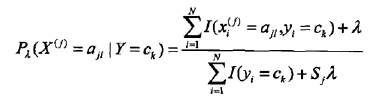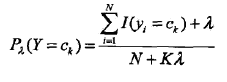朴素贝叶斯法本文是《统计学习方法》第4章的笔记，用图形补充说明了条件概率分布计算时可能引发的维数灾难，在文末用Python实现了一个基于贝叶斯文本分类器的简单情感极性分析器，可以分析中文句子的情感极性。

朴素贝叶斯法的学习与分类

基本方法豆知识：维数灾难

dimensionality = 10
print 1 / (0.01 ** dimensionality)

# -*- coding:utf-8 -*-
# Filename: dimensionality.py
# Author：hankcs
# Date: 2015/2/6 14:40
from matplotlib import pyplot as plt
import numpy as np

max_dimensionality = 10
ax = plt.axes(xlim=(0, max_dimensionality), ylim=(0, 1 / (0.01 ** max_dimensionality)))
x = np.linspace(0, max_dimensionality, 1000)
y = 1 / (0.01 ** x)
plt.plot(x, y, lw=2)
plt.show()后验概率最大化的含义f(X)就是分类器的决策函数，损失函数的参数其实是一个联合分布。Ex∑[L()]P(ck|X)=P(X)∑[L()]P(X,ck)/P(X)=∑[L()]P(X,ck)=E[L()]朴素贝叶斯法的参数估计

极大似然估计学习与分类算法1、条件概率的贝叶斯估计：2、先验概率的贝叶斯估计：贝叶斯情感极性分析器

# -*- coding:utf-8 -*-
# Filename: Bayes.py
# Author：hankcs
# Date: 2015/2/6 22:25
from math import log, exp

class LaplaceEstimate(object):
"""
拉普拉斯平滑处理的贝叶斯估计
"""

def __init__(self):
self.d = {}  # [词-词频]的map
self.total = 0.0  # 全部词的词频
self.none = 1  # 当一个词不存在的时候，它的词频（等于0+1）

def exists(self, key):
return key in self.d

def getsum(self):
return self.total

def get(self, key):
if not self.exists(key):
return False, self.none
return True, self.d[key]

def getprob(self, key):
"""
估计先验概率
:param key: 词
:return: 概率
"""
return float(self.get(key)) / self.total

def samples(self):
"""
获取全部样本
:return:
"""
return self.d.keys()

def add(self, key, value):
self.total += value
if not self.exists(key):
self.d[key] = 1
self.total += 1
self.d[key] += value

class Bayes(object):
def __init__(self):
self.d = {}  # [标签, 概率] map
self.total = 0  # 全部词频

def train(self, data):
for d in data:  # d是[[词链表], 标签]
c = d  # c是分类
if c not in self.d:
self.d[c] = LaplaceEstimate()  # d[c]是概率统计工具
for word in d:
self.d[c].add(word, 1)  # 统计词频
self.total = sum(map(lambda x: self.d[x].getsum(), self.d.keys()))

def classify(self, x):
tmp = {}
for c in self.d:  # 分类
tmp[c] = log(self.d[c].getsum()) - log(self.total)  # P(Y=ck)
for word in x:
tmp[c] += log(self.d[c].getprob(word))          # P(Xj=xj | Y=ck)
ret, prob = 0, 0
for c in self.d:
now = 0
try:
for otherc in self.d:
now += exp(tmp[otherc] - tmp[c])            # 将对数还原为1/p
now = 1 / now
except OverflowError:
now = 0
if now > prob:
ret, prob = c, now
return (ret, prob)

class Sentiment(object):
def __init__(self):
self.classifier = Bayes()

def segment(self, sent):
words = sent.split(' ')
return words

def train(self, neg_docs, pos_docs):
data = []
for sent in neg_docs:
data.append([self.segment(sent), u'neg'])
for sent in pos_docs:
data.append([self.segment(sent), u'pos'])
self.classifier.train(data)

def classify(self, sent):

return self.classifier.classify(self.segment(sent))

s = Sentiment()
s.train([u'糟糕', u'好 差劲'], [u'优秀', u'很 好']) # 空格分词

print s.classify(u"好 优秀")

(u'pos', 0.6666666666666665)

Reference

评论 17

1. #11

博主，你好，给出的样例 print s.classify(u”好 优秀”)，这个结果应该错了吧，两个正负面训练集数据词频相同，“优秀”虽然不在负面训练集中，但经过拉普拉斯平滑之后，词频为1，所以最终的正负面概率应该是相等的??

while true9个月前 (01-16)回复
• 45行、48行是否重复加？

while true9个月前 (01-16)回复
2. #10

def classify(self, x):
tmp = {}
for c in self.d: # 分类
tmp[c] = log(self.d[c].getsum()) – log(self.total) # P(Y=ck)
for word in x:
tmp[c] += log(self.d[c].getprob(word)) #log(关于不同的xj累乘( P(Xj=xj | Y=ck))*P(Y=ck))=log(P(X|Y=ck)*P(Y=ck))=log(P(X,Y=ck))
ret, prob = 0, 0
for c in self.d:
now = 0
try:
for otherc in self.d:
now += exp(tmp[otherc] – tmp[c]) # (关于不同的Y取值ck累加(P(x|Y=ck)*P(Y=ck)))|P(x|Y=ck)*P(Y=ck)=P(X)/P(x,Y=ck)=1/P(Y=ck|X)
now = 1 / now #将对数还原为p(Y=ck|X)
except OverflowError:
now = 0
if now > prob: #不断更新最大概率的类别
ret, prob = c, now
return (ret, prob)
我自己的理解， 不知道对不对

yan_h_y1年前 (2018-07-18)回复
3. #9

解释一下博主的第74-83行判断分类的代码。
确实可以直接通过直接比较tmp[c]的大小直接判断label。
原代码中的now>prob就是用来寻找最大概率的。
77-81行是用来还原对数得到真实后验概率的，考虑了原公式中分母中的全概率。
不考虑全概率得不到后验概率。
now+=exp(tmp[otherc]-tmp[c])
当otherc=c时，这部分为1。即本身对其本身来说为1。
当otherc!=c时，这部分为x。即otherc对于c来说的相对大小。假设为2。
则判断为标记c的概率为1/3。

Su_Chi1年前 (2018-07-15)回复
4. #8

博主你好，请教一下，对于朴素贝叶斯问题，可以直接使用最大化后验概率来对给定验证集进行预测；当如果使用最大化后验概率求解问题存在难度时，就可以通过贝叶斯估计分别求出先验概率和条件概率，然后在求解后验概率。这么理解正确吗？也就是说，《统计学习方法》的朴素贝叶斯法这一章中提供两种求解方案？

jozee1年前 (2018-06-03)回复
5. #7

classify的对数和指数不是为了归一化吧，我记得应该是防止下溢，由于有浮点数计算才用的对数和指数，博主能给解释一下吗？

哈哈哈1年前 (2018-05-17)回复
6. #6

代码74-83这一段是什么意思呢？

LIncoLN2年前 (2017-08-03)回复
• 这段搞懂了，这是按照原始的朴素贝叶斯基本公式来做的，但是实际上只需要比较tmp[c]的大小就好了，因为对所有的c，朴素贝叶斯基本公式的分母都是一样的

LIncoLN2年前 (2017-08-03)回复
7. #5

推出来了。。博主，我刚刚开始研究ml，公式能看懂，但是转化成代码，有些困难，希望你可以给一些建议~

yeepom4年前 (2016-04-28)回复
• 能解释下么

LIncoLN2年前 (2017-08-03)回复
8. #4

博主，代码里面第78行，是如何推导的啊？推了好久，没弄明白。。

yeepom4年前 (2016-04-28)回复
9. #3

请问，怎么对文本信息进行分类

Dalian5年前 (2015-03-30)回复
10. #2

s = Sentiment()

hankcs5年前 (2015-03-27)回复
11. #1

我想知道的是楼主学概率论和高数么？明明是文科生为什么这些公式理解起来这么轻松啊。。

lzru--5年前 (2015-02-11)回复
• 学是学过，早忘光了，基本现学的。我是个怪物。

hankcs5年前 (2015-02-12)回复